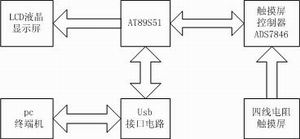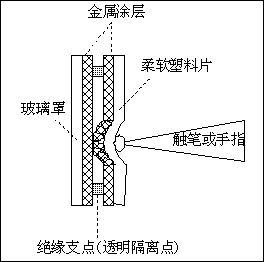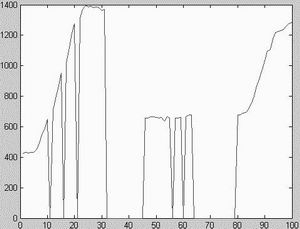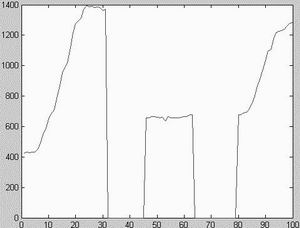# Online dynamic signature authentication system based on ADS7846

PickÂ Â  want:Â Â Â Â Â Â  This article focuses on how to use AT89S51 , touch screen and touch screen controller ADS7846 to realize online dynamic signature 3D data collection and preprocessing.

Because the touch screen is convenient for input, thin and easy to carry, more and more electronic products now use the touch screen as the input device of the man-machine interface. In dynamic signature authentication, a touch screen is also used as an input device for data collection of handwriting.

The system is mainly composed of four-wire resistive touch screen, touch screen controller ADS7846, and single-chip 89S51 . The system block diagram is shown as in Fig. 1 .

Four-wire resistive touch screen

The resistive touch screen adopts resistive analog technology. It uses a layer of glass as the base layer, coated with a transparent metal oxide (ITO indium oxide ) conductive layer, and covered with a layer of glass or a smooth plastic layer hardened on the outer surface, and its inner surface is also coated with a layer of ITO Conductive layer, there are many small transparent isolation points between them to separate the two conductive layers and insulate them. Whenever a pen or finger presses down, the two conductive layers touch each other, thus forming a loop ( Figure 2) .

Both ends of the conductive layer are coated with a silver glue called a pair of electrodes on the working surface. One of the upper and lower conductive layers is horizontal and the other is vertical. They are used to measure the coordinate positions of X and Y respectively . The electrodes on the horizontal plane are called X+ electrodes and X- electrodes, and the electrodes on the vertical plane are called Y+ electrodes and Y- electrodes. When working, the two electrodes provide a reference voltage according to the measurement needs or are used as measuring terminals to measure the position of the contact point. When measuring the X coordinate of the contact point , the X+ electrode and X- electrode on the conductive layer are respectively connected to the reference voltage and If there is no voltage applied to the Y electrode, a uniform voltage distribution will be formed between the X electrodes. Using the Y+ electrode as the measurement point, the voltage value obtained can be converted by A/D to determine the X coordinate of the contact point . The Y coordinate is also similar, just change it to apply voltage to the Y electrode and no voltage to the X electrode.

The principle of measuring the pressure of touch is related to the characteristics of ITO ( indium oxide ) material. The conductivity formula of ITO conductive film is r=r0(1+l0/d) , where d is the thickness of the conductive film, so the conductivity of ITO It is inversely proportional to the thickness of the ITO conductive film, so when the pressure of the touch is greater, the ITO conductive film at the contact point is thinner, so the corresponding conductivity is larger, and the resistance is smaller. Therefore, the measurement of touch pressure is essentially a measurement of contact The resistance value of the ITO conductive film.Â Â Â Â

ADS7846 is a digital-to-analog conversion chip produced by BURN-BROWN Company for four-wire resistive touch screen. Inside there is a measuring circuit network composed of multi-channel analog switches and a 12 -bit A/D converter. When working, the 7846 opens the corresponding switch channel according to the different command words received by the data input port DIN , and accepts the returned analog voltage, obtains the corresponding digital quantity through A/D conversion, and then transmits it back to the single-chip microcomputer through DOUT .

AT89S51 is an upgraded product of 89C51 newly launched by ATMEL .

The communication between AT89S51 and ADS7846 is mainly carried out through the I/O port of the one- chip computer and the five pins of CLK , CS , DOUT , DIN and BUSY of 7846 . When the system is working, the microcontroller first selects 7846 through the chip selection signal , and then sends the command control word data to 7846 through DIN , and matches the corresponding timing pulse through the CLK pin. When the BUSY bit is set, it passes through the DOUT data output port Get the 12 -bit conversion result.

Since the system is required to collect three-dimensional data including X coordinate, Y coordinate and pressure, first send the command word to open the measurement X channel, the returned digital value is the X coordinate, and then send the command word to open the measurement Y channel. The obtained value is the Y coordinate. Then get the values â€‹â€‹of Z1 and Z2 in turn , and use the conversion formula

RTOUCH

You can calculate RTOUCH, which is the value of pressure Z ( where RX-plant is the x- axis resistance value, which is related to the performance parameters of the touch screen, and X-PosiTIon is the X- coordinate value obtained at the beginning ) .

It is worth mentioning here that Z1 and Z2 are still 12 -bit A/D converted digital values â€‹â€‹after conversion, but the Z value obtained by the conversion formula is actually the resistance value of the two ITO oxide films between the contact points . This completes the three-dimensional information collection at a certain moment. According to actual requirements, use the timer to set the sampling time to 10ms . The collected data is sent to the PC through the USB interface to be saved for subsequent matching processing. At the same time, the collected data can be displayed on the LCD screen for observation while writing.Figure 1Â  System circuit block diagramPicture 2Â  Schematic diagram of the touch screenFigure 3 Data waveform before processingFigure 4 Data waveform after processing

Initial calibration of touch screen

Because some edge parts of the touch screen are invalid touch areas, the actual converted X and Y coordinates are not from 0 to 4095 , so initial calibration is required, and the entire range of 0 to 4095 is linearized through the conversion formula , the conversion formula for the X-coordinate value X = (X-Xmin) & TImes; 4095 / (Xmax-Xmin), wherein Xmax and Xmin are the maximum value and the minimum value of effective area in the X direction touchscreen touch. Similarly, the conversion formula corresponding to the Y coordinate value is Y=(Y-Ymin) &TImes; 4095/(Ymax-Ymin) , where Ymax and Ymin are the maximum and minimum values â€‹â€‹of the effective touch area of â€‹â€‹the touch screen in the Y direction, respectively . The pressure value represents the size of the resistance value, and can not directly reflect the size of the pressure. Therefore, according to the actual needs, it is converted into a pressure value of 0 to 256 corresponding to the size of the pressure. The conversion formula is Z=(Zmax-Z) &TImes; 256/(Zmax-Zmin) , where Zmax and Zmin are respectively the maximum resistance value and the minimum resistance value when the pen is in contact with the touch screen.

The experimental data shows that the characteristic parameters of the touch screen used are: Xmax and Xmin are 3860 and 180 , respectively ; Zmax and Zmin are 3690 and 440 , and 540 and 350 , respectively . Since the physical characteristics of each touch screen and writing pen are different, the system must re-initialize and calibrate each time the touch screen or writing pen is replaced.

Data preprocessing

In the process of data collection, there are many factors that affect the accuracy of the data, so some corresponding preprocessing must be done to the data to ensure the accuracy of the collected data. There are mainly the following factors:

Missing points caused by writing jitter

During data collection, zero points will appear in the continuous data. There are two possibilities for zero points: one is caused by the change of strokes during normal writing, and the other is because the touch screen is not flat enough or when writing. Jitter is caused by the discontinuity of handwriting. The method of difference is mainly to look at the discontinuity time, which is the number of consecutive occurrences of zero values. Generally, the discontinuity time caused by jitter is less than the set sampling time of 10ms , so the zero value generated by jitter is between two Only one valid data can appear in the middle, which can be considered as an invalid zero value, and the occurrence of two or more consecutive zero values â€‹â€‹can be considered as a valid zero value generated by a stroke change without processing. When a certain zero value is judged After the invalid zero value, linear interpolation is performed to compensate for the loss of data, that is, an=(an+1+an-1)/2 , where an is the invalid zero point , and an-1 and an+1 are an respectively A valid value before and after. Figures 3 and 4 are waveforms before and after treatment, respectively.

Removal of repeated collection points

Due to the writerâ€™s pause while writing, repeated collections at a certain point are caused. Obviously, there will be a large error with the sample data in the subsequent matching. Therefore, it is necessary to delete the repeated data. Whenever the two points before and after are judged When the X and Y coordinates are the same, it can be concluded that they are duplicate points, then the latter point is deleted, and the subsequent points are used to fill in. This ensures that the collected data can truly reflect the handwriting information.

Calibration of writing position

The point at which the writer starts the pen each time he writes is not necessarily the same. In this way, the result of each writing sample has a constant difference from the sample value in the sample template. Therefore, it is necessary to calibrate the writing position. The processing method is to X and Y coordinates of the sample data collected with the first data in the X and Y coordinates of the first comparison data, formulas are DX = (X1-X '1 ), DY = (Y1-Y' 1) , where X1 and Y1 are the X coordinate value and Y coordinate value of the first point of the sampled data ,Â  X ' 1 and Y ' 1 are the X coordinate value and Y coordinate value of the first point in the sample data ,Â  DX and DY are the offset values between the sampled data and the sample data in the X and Y directions. Therefore, the calibration formula is: Xn=Xn+DX , Yn=Yn+DY .

The above are several methods for preprocessing the data. Preprocessing can provide more accurate data for subsequent handwriting matching and reduce the amount of matching calculations. Therefore, preprocessing is an important step before handwriting matching authentication. Directly affect the accuracy of certification.

Phenolic Paper Laminated Sheet

Phenolic paper laminated sheet is a hard, dense material made by applying heat and pressure to layers of paper or fabric, impregnated with a synthetic resin. These phenolics are approx half the weight of aluminum and their physical toughness resists abrasion, friction, impact, and material fatigue. Special properties fight off the corrosive action of water and many chemicals, increasing the service life and efficiency of your product parts. The insulating qualities of each grade, such as low dissipation factor and excellent dielectric strength, make them valuable for many precise electrical and electronic applications.
It is made of insulating impregnated paper impregnated with phenolic resin and baked and hot pressed. Has high mechanical properties. The heat resistance level is E level.

Phenolic Paper,Phenolic Cardboard,Paper Phenolic Sheet,Phenolic Paper Laminated Sheet

Yingkou Dongyuan Electrical Insulation Board Co.,Ltd , https://www.dy-insulation.com